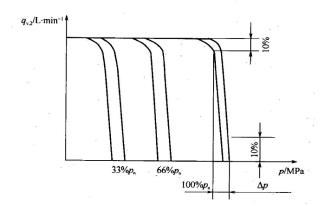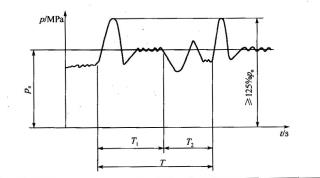Created on：2021-05-27 14:45

# Test items and methods of axial piston pump

Test items and methods of axial piston pump

c. Type test. Type test items and test methods shall be in accordance with the provisions of schedule 28.

Type test items and methods of axial piston pump

 Serial number Test items test method remarks one Discharge verification test According to GB 7936 two Efficiency test a) Under the maximum displacement and rated speed, the outlet pressure of the tested pump is gradually increased to 25% of the rated pressure. After the test state is stable, the data related to efficiency is measured b) According to the above method, when the outlet pressure of the tested pump is 40%, 55%, 70%, 80% and 100% of the rated pressure, the data related to the efficiency are measured respectively c) When the speed is about 1000 / <, 85%, 70%, 55%, 40%, 30%, 20% and 10% of the rated speed, the data of the tested pump and efficiency are measured respectively at the above test pressure points d) Draw the performance curve (see Fig. 19) e) The volumetric efficiency of the tested pump was measured at least six equal pressure points in the range of no-load pressure to rated pressure when the inlet oil temperature was 20 ~ 35 ℃ and 70 ~ 80 ℃ at rated speed f) The characteristic curves of efficiency, flow rate and power varying with pressure are drawn a) (b) the pressure value calculated according to the percentage in two articles shall be rounded to 1MPa; c) The speed value calculated by percentage in the article is rounded to 10R / min three Variable characteristic test a) Constant power variable displacement pump Determination of the lowest pressure transition point: adjust the variable mechanism to make the tested pump in the lowest pressure transition state, and measure the outlet pressure of the tested pump Determination of the maximum pressure transition point: adjust the variable mechanism to make the tested pump in the maximum pressure transition state, and measure the pump outlet pressure Measurement of constant power characteristics: adjust the variable mechanism according to the design requirements, measure the corresponding data of pressure and flow, draw the constant power characteristic curve (pressure flow characteristic curve) (see Fig. 21) and test other characteristics according to the design requirements b) Constant pressure variable displacement pump Constant pressure static characteristic test: load under the maximum displacement and rated speed, draw the flow pressure characteristic line under different set pressure, as shown in the figure below Setting pressure: 33% PN, 66% PN, 100% PN Output flow: 0 ～ 100% QV, 2Note: 1. Up and down curves shall not be less than 10 points 2. The safety valve in the test system shall not be opened 3. PN is the rated pressure c) Other variable displacement pumps Test according to design requirements or user requirements Variable displacement pump to do the test four Self priming test Under the working conditions of maximum displacement, rated speed and no-load pressure, the displacement of the pump with zero suction vacuum was measured. On this basis, gradually increase the suction resistance until the displacement decreases by 1%, and measure the vacuum degree Self priming pump to do the test five Noise test Under the maximum displacement, set speed and inlet pressure of 0.1MPa absolute pressure, the noise values of at least six equal pressure points within the range of no-load pressure to rated pressure of the tested pump were measured respectively When the rated speed is greater than or equal to 1500r / min, the set speed is 1500r / min; when 1000r / min ≤ rated speed < 1500r / min, the set speed is 1000r / min; when rated speed < 1000r / min, the set speed is rated speed The background noise should be more than 10dB (a) lower than the measured noise of the tested pump, otherwise it should be corrected. This project is an examination project six Low temperature test Make the test pump and inlet oil temperature at - 20 ~ 15 ℃, the oil viscosity within the maximum viscosity range allowed by the test pump, and start the test pump at least five times under rated speed and no-load pressure condition (the variable displacement pump is at the maximum displacement) a) Do this test when required; b) It can be negotiated by the manufacturer and the user in industrial application seven High temperature test Under the rated condition, the inlet oil temperature is 90 ~ 100 ℃, the oil viscosity is not lower than the minimum allowable viscosity of the pump under test, and the pump runs continuously for more than 1 hour eight Overspeed test When the speed is 115% of the rated speed (the variable displacement pump is at the maximum displacement), the pump is continuously operated at no-load pressure and rated pressure for more than 15 minutes, and the inlet oil temperature of the tested pump is 30 ~ 60 ℃ nine Overload test Under the working condition of rated speed, maximum pressure or 125% of rated pressure (select the higher one, the variable displacement pump is at the maximum displacement), the pump operates continuously. During the test, the inlet oil temperature of the tested pump is 30 ~ 60 ℃, and the test time shall comply with the provisions of item ② m in (5) ten impact test a) Quantitative and manual variable displacement pumps The pressure impact test was carried out under the maximum displacement and rated speed. The impact frequency is 10-30 times / mln, the impact waveform is in accordance with FIG. 22, continuous operation b) Constant power variable displacement pump Under the constant power characteristic of 40% rated power and rated speed, the pressure impact test is carried out. The impact frequency is 10-30 times / min, and the impact waveform conforms to Fig. 22. The test runs continuously c) The constant pressure impulse (step) cycle test is carried out continuously when the rated speed and flow of the constant pressure variable displacement pump are between Lo% qvmax ≤ QV ≤ 80% qvmax. The waveform is shown in the figure belowT -- impact cycle period; T1 -- pressure holding time of rated pressure and small flow rate; T2 -- pressure holding time of rated pressure and large flow rate; PN -- rated pressure; Qvmax -- maximum flow d) Other variable types Test according to variable characteristics of maximum power or user requirements During the impact test, the inlet oil temperature of the tested pump is 30 ~ 60 ℃, and the test times shall comply with the provisions of item ② m in (5) Record the shock wave eleven Full load test Under the rated working condition, the pump under test operates continuously when the inlet oil temperature is 30 ~ 60 ℃, and the test time shall comply with the provisions of item ② m in (5) twelve Efficiency test After the above tests, the volumetric efficiency and total efficiency under rated conditions were measured thirteen Sealing performance inspection Wipe the tested pump clean. If some parts cannot be wiped clean at one time and "false" leakage occurs after operation, it is allowed to wipe clean again   a)静密封：将干净吸水纸压贴于静密封部位，然后取下，纸上如有油迹即为渗油   b)动密封：在动密封部位下方放置白纸，于规定时间内纸上不应有油滴

Note: 1. Continuous operation test time or times refers to the cumulative value after deducting the failure time or times unrelated to the tested pump.

2. Test items 9-11 belong to durability test items.

④ The expression method of test data and results is the same as that of gear pump.

(7) Inspection method of assembly and appearance. Same as gear pump.

(8) Inspection rules. Same as gear pump.

(9) Marking and packaging. Same as gear pump.

Home    Article    Test items and methods of axial piston pump